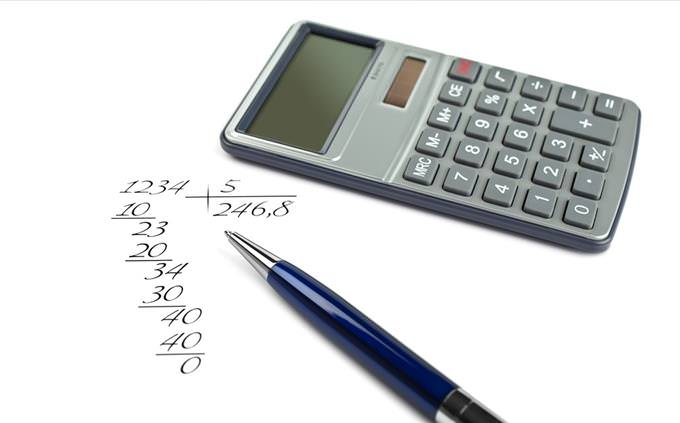# Math Quiz: Can You Solve These 12 Tricky Problems?

A+ A-
Welcome to another BabaMail math quiz! We have some really creative problems for you to solve, 12 in number, and increasing in difficulty. None require a calculator if you give it some thought, and look in the end at the results to see where you went wrong and why! We offer full solutions in case you got it wrong. Good luck!What is the next number in this series: 9, 19, 21, 32, 34, X?
The series goes up 10, down 2, then up 11, down 2, meaning the next will be up 12 - 34 + 12 = 46
29
31
44
46When throwing 2 dice, what are the chances of getting a 12?
1/6 times 1/6 for each dice = 1/36
1 in 12
1 in 24
1 in 36
1 in 48How many 7s there are between 1 - 100?
40
20
35
15The King Has 5 Daughters, each one is half the age of the other. If the king met the mother of the oldest 40 years ago, what is the age of his youngest daughter?
There are a few possibilities IF there was no age cap when the oldest was born. Since she was born less than 40 years ago, then the sequence must be 2,4,8,16,32 - meaning the youngest is 2 years old.
3
6
1
2There are 12 identical Coins. They all have the exact same weight except for 1 coin that is heavier than the other 11 coins. How many weighings, at the minimum, are required to identify this different coin?
(3 weights - 4 and 4 coins, 2 and 2, 1 and 1)
4
5
6
3What 3 Consecutive Numbers can you Multiply to get 4080?
15x16x17
12x13x14
21x20x19
18x19x20You Need to Get to 1000, but you can only add numbers that are all eights (8,88.. etc). How many different numbers would you need?
888+88+8+8+8
3
4
5
6What's the missing number: 11x11=4, 22x22=16, 33x33=36, 55x55=X?
72
56
100
116Use four 9s (any way you want) to get to 100 - How many different numbers would you need?
99 + (9/9) = 100
3
4
2
Can't be doneMarch = 538, February = 8210, July = 4711, April 549, September=?
(first digit is the number of letters, 2nd the number of the month, and third is the first two numbers combined so the answer is 9918)
9918
9219
5611
8918142 = 0, 3271 = 0, 6211 = 1, 83461 = 3, 908763 = 5, 4789033 = ?
You count the number of circles in the numbers to get the answer.
2
6
9
4What the missing number? 11x23=10, 35x13=32, 41x72=45, 62x51=X?
The formula here is (a+b x c+d)
48
64
17
113
Oops! You Got a Low Score...Maybe you're not focused or maybe you haven't done math like this in a long time. It could also be you haven't let yourself enough time to really think about it. Why not try again, and if not - have a look at our explanations of your mistakes below.
You Did Great!You didn't ace the test, but you got high marks! This isn't easy to do considering few of us actually use math like this in our every day life. Kudos for keeping the math alive and your brain well and functioning, and have a look below at your mistakes to learn from them.